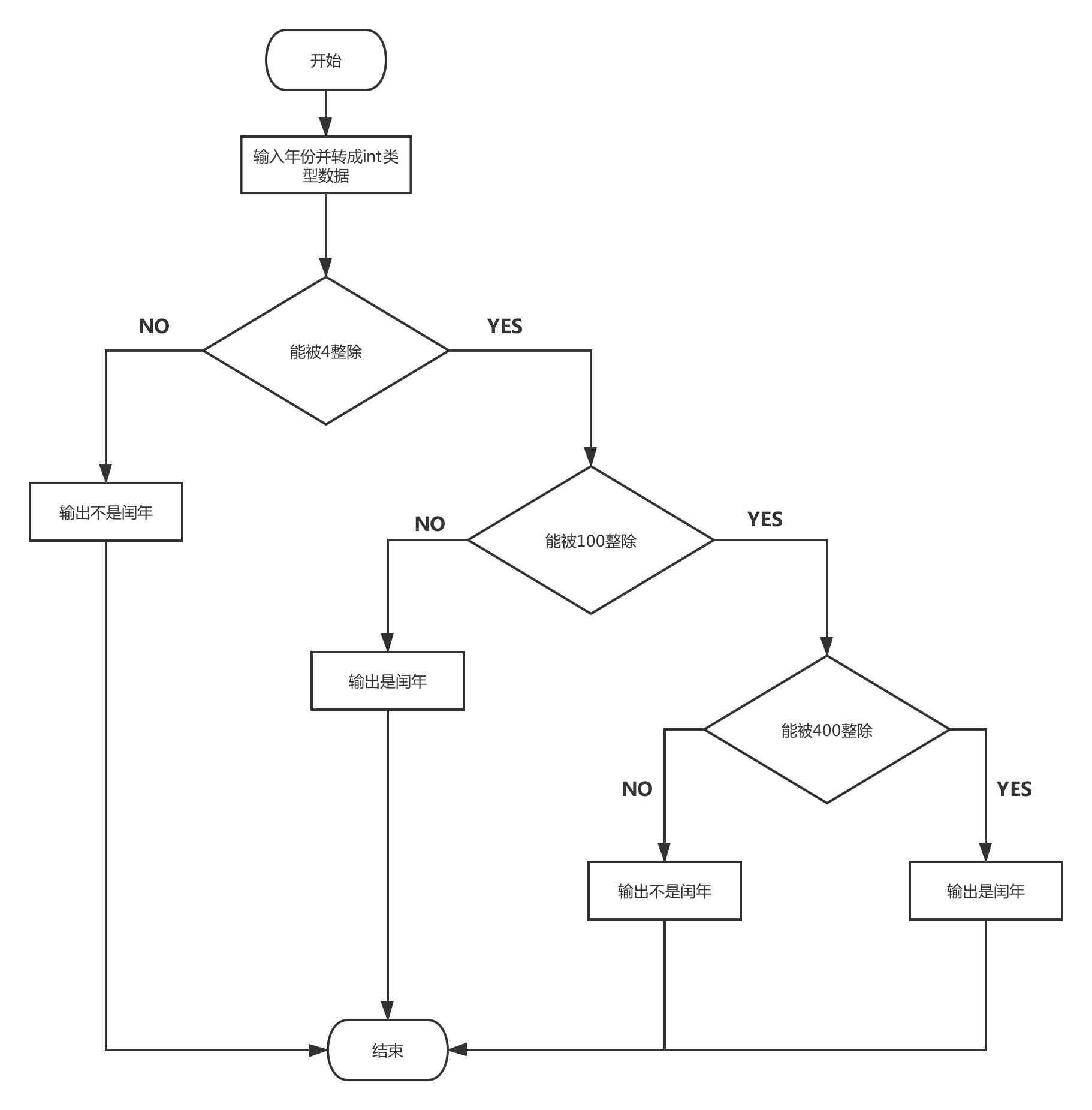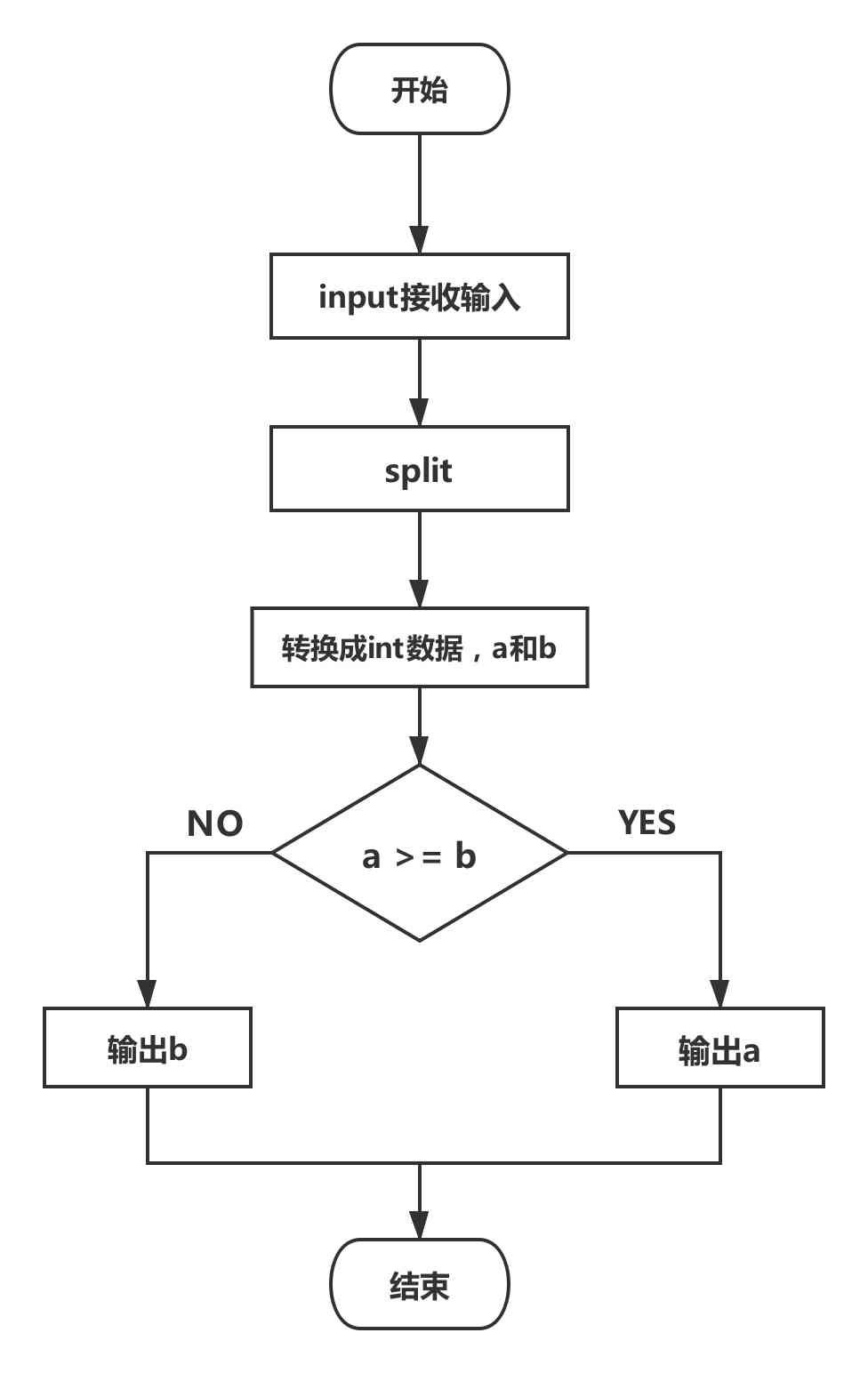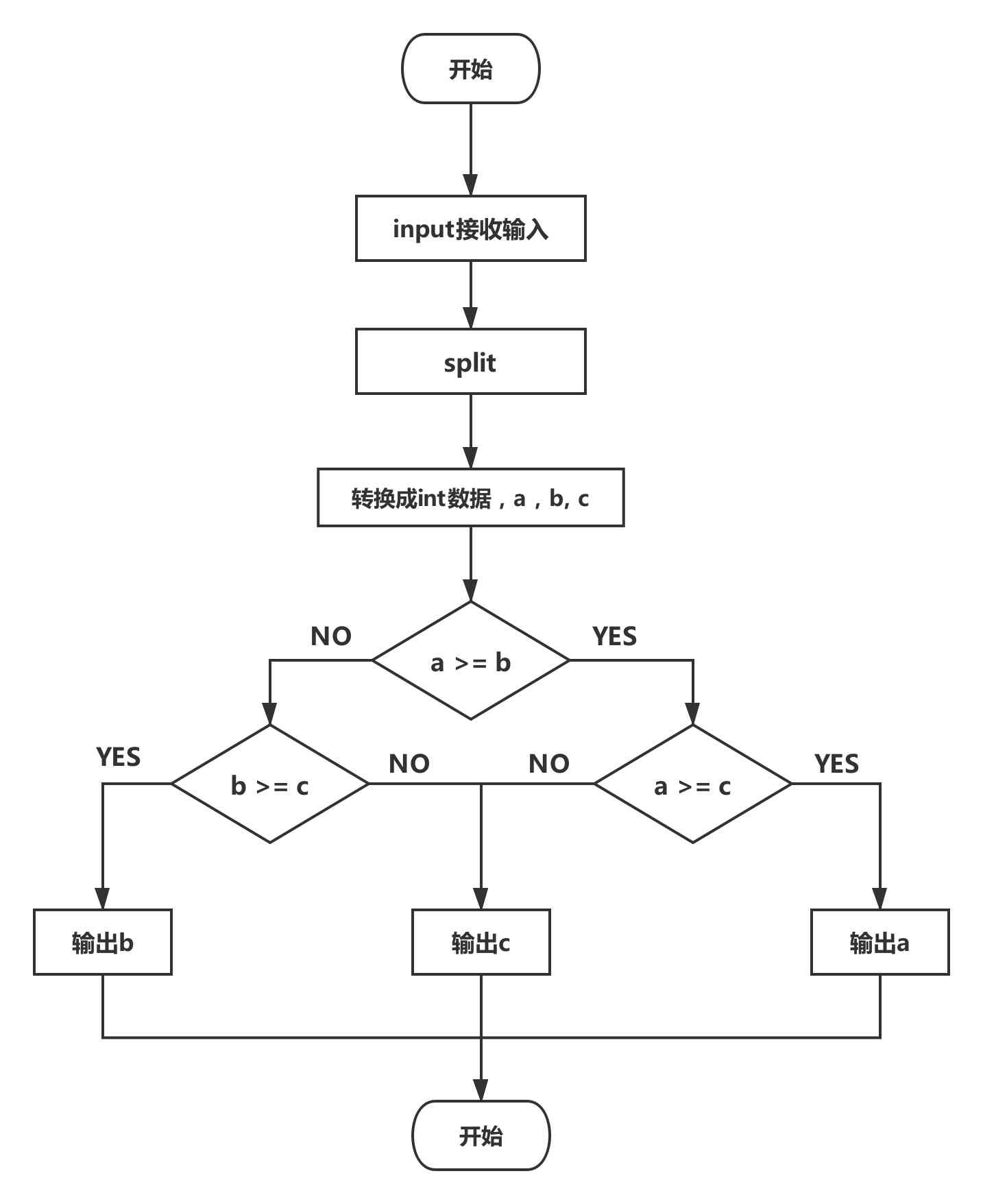# 条件控制练习题

## 1.判断日期是否合法

### 1.1 题目要求

``````输入: 3月15日

``````

``````输入: 5月32日

``````

### 1.2 思路分析

1，3，5，7，8，10，12月有31天，非闰年2月份有28天，其他月份有30天。

1. 使用字符串replace方法将“日”替换成空字符串
2. 使用split方法，“月”作为分割符对字符串进行分割，得到列表
3. 将列表里的数据转换成int类型数据

### 1.3 示例代码

``````date_time = input("请输入月份和日期：")
date_time = date_time.replace("日", '')
time_lst = date_time.split('月')
month, day = int(time_lst), int(time_lst)

if month in (1, 3, 5, 7, 8, 10, 12):
if 1 <= day <= 31:
print("合法")
else:
print("不合法")
elif month == 2:
if 1 <= day <= 28:
print("合法")
else:
print("不合法")
else:
if 1 <= day <= 30:
print("合法")
else:
print("不合法")
``````

### 1.4 程序流程图### 1.5 更简洁的写法

``````date_time = input("请输入月份和日期：")
date_time = date_time.replace("日", '')
time_lst = date_time.split('月')
month, day = int(time_lst), int(time_lst)
min_day = 1
max_day = 30

if month in (1, 3, 5, 7, 8, 10, 12):
max_day = 31
elif month == 2:
max_day = 28

if min_day <= day <= max_day:
print("合法")
else:
print("不合法")
``````

## 2. 判断闰年

### 2.1 题目要求

1. 不能被4整除的一定不是闰年
2. 如果年份能被4整除且不能被100整除，则是闰年
3. 如果年份能被4整除，同时又能被100整除，那么要判断它能否被400整除，如果可以则是闰年，否则不是

### 2.2 示例代码

``````year = int(input("请输入一个年份："))

if (year % 4) == 0:
if year % 100 == 0:
if year % 400 == 0:
print("{year} 是闰年".format(year=year))
else:
print("{year} 不是闰年".format(year=year))
else:
print("{year} 是闰年".format(year=year))
else:
print("{year} 不是闰年".format(year=year))
``````

### 2.3 程序流程图## 3. 比较两个数大小

### 3.2 思路分析

input函数返回的数据是一个字符串，使用字符串split方法可以将字符串解析成一个列表，列表里有两个字符串，将他们转换成int类型数据，分别赋值给变量a,b，对这两个数值进行比较，输出最大的一个

### 3.3 示例代码

``````value = input("请输入两个整数,中间用空格分开:")
lst = value.split()
a, b = int(lst), int(lst)
if a >= b:
print(a)
else:
print(b)
``````

### 3.4 程序流程图## 4. 比较三个数大小

### 4.2 思路分析### 4.3 示例代码

``````value = input("请输入两个整数,中间用空格分开:")
lst = value.split()
a, b, c = int(lst), int(lst), int(lst)

if a >= b:
if a >= c:
print(a)
else:
print(c)
else:
if b >= c:
print(b)
else:
print(c)
``````

### 4.4 另一种算法

4.3 的代码，并不是一种好的算法，逻辑处理过于繁琐，作为训练使用还可以，若是用于实际工作，空拍就要被笑话了,下面提供一种更好的算法

``````value = input("请输入两个整数,中间用空格分开:")
lst = value.split()
a, b, c = int(lst), int(lst), int(lst)

max_value = a
if b >= max_value:
max_value = b

if c >= max_value:
max_value = c

print(max_value)
``````QQ交流群: 211426309